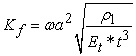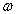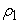﻿ Free Vibration Analysis of Clamped Skew Composite Plate Using Finite Element MethodPublications are Open
Access in this journal
Article Versions
Export Article
• Normal Style
• MLA Style
• APA Style
• Chicago Style
Research Article
Open Access Peer-reviewed

Free Vibration Analysis of Clamped Skew Composite Plate Using Finite Element Method

American Journal of Mechanical Engineering. 2021, 9(1), 41-56. DOI: 10.12691/ajme-9-1-5
Received September 03, 2021; Revised October 19, 2021; Accepted October 28, 2021

Abstract

This study is presented of two models were built to simulate the skew composite plates and these models are SHELL281 and SOLID186 element. Three types of laminates arrangement were used to study the effect of skew angle, fiber orientation and length-to-width ratio on the first five natural frequencies of the clamped composite plates. The skew angle was changed from (0°) to (75°) with (15°) steps while the fiber orientation was changed from (0°) to (90°) with (15°) steps and the length-to-width ratio was (0.5, 1, 1.25, 1.5 and 2). The results showed that the first five frequency parameters increase for three layouts when the skew angle increases at constant fiber orientation and constant length-to-width ratio. Also, the fiber orientation has a slight effect on the first five frequency parameters in (0/β/0) layout. The effect of length-to-width ratio on the first five frequency parameters depends on the skew angle (smaller or larger than (60o)), fiber orientation and the laminates layout. Finally, the values of the first five frequency parameters of (β/0/β) and (β/β/β) layouts are close to each other.

1. Introduction

Composite materials can be defined as a combination of two or more materials in a macroscopic scale by mechanical and chemical bonding in order to produce a new material that has desired mechanical properties. Because of increasing strength-to-weight ratio and specific modulus, composite plates are widely used in the manufacturing of structural members. The laminated composite plate is a kind of composite materials applications and it is used commonly in automobiles, robotics, day to day appliances, building industry, aerospace structures, nuclear structures, marine vehicles and ships. The laminated composite skew plates are also used in these applications and the analysis of this type of composite plate is very difficult for different reasons such as increased number of material constants, increased number of layers and different structural couplings. Due to these difficulties, the exact mathematical solutions of the free vibration problems of skew composite plates are not available, therefore, the approximate methods are used to analyze the free vibration problem.

In the last few decades, many researchers investigated extensively the free vibration behavior of skew plates. The finite element method is one of the methods used to analyze the free vibration problem of the skew composite plates. 1 used method to estimate the natural frequencies of simply-supported rhombic plate. For clamped rhombic plates, 2 used FEM to obtain natural frequencies. 3 studied mode shapes and natural frequencies of skew plates with clamped boundary condition by adopting Galerkin FEM. 4 calculated the modes of free-free CFRP plates considering the effect of transverse shear deformation using the finite element method. 5 used finite element method to study the effect of skew angles, fiber orientation and boundary conditions on the vibration behavior of rhombic orthotropic plates. 6 used a Co finite element method to investigate the free vibration problem of sandwich and laminated beam and plate. 7 calculated mode shapes and natural frequencies of isotropic skew plates by adopting the hierarchical finite element method. 8 determined (a) the effects of skew angle, (b) angle of fiber orientation, (c) aspect ratio (length-to-width ratio) and (d) length-to-thickness ratio on the natural frequency of one lamina glass/epoxy and graphite/epoxy skew plates. 9 combined the Mindlin plate theory and the finite element method to study the effect of partially supported boundaries on the free vibration behavior of right triangular orthotropic plates. 10 used C0 continuous isoparametric quadrilateral element to study the free vibration of thin and thick cantilever skew plates using. 11, 12 used finite element method to study the free vibration problem of the deep cylindrical panel and thin isotropic conical panels. 13 studied the free vibration of laminated skew plates using a general high precision triangular bending element. 14 used a nine-node degenerated shell element to analyze the free vibration of isotropic plates based on the first-order shear deformation theory. 15 used FEM to study the free vibrations problem of skew composite plates with large amplitude. 16 developed a new finite element model to simulate anisotropic and laminated doubly curved moderately thick composite shell panels using the first-order shear deformation theory. 17 Used isoparametric finite element model for studying the free vibration behavior of different types of skew plates. 18 studied the static and free vibration of general shell structures using the element-free Galerkin method. 19 used a higher-order finite element to analyze the free vibration problem of FG curved panels. 20 used finite element method to study flexural natural frequencies of simply-supported (SSSS) and clamped (CCCC) skew plates assuming isotropic and laminated composite materials. They studied the effects of aspect ratio, length-to-thickness-ratio and the skew angle the natural frequency of isotropic skew plates while they studied the additional factors such as fiber orientation angle and numbers of layers. They used these factors to compare between CQUAD4 element and CQUAD8 element and studied the accuracy of their results. 21 investigated experimentally the free vibration of skew laminated plates and compared between experimental and finite element results. 22 used ANSYS APDL software to study free vibration behaviour of rectangular composite laminate plate for different boundary conditions. He studied the effects of modulus ratios side-to-thickness ratios and aspect ratios on the first five natural frequencies of plate for different boundary conditions. 23 used a commercial finite element program (ANSYS 14.5) to compute the natural frequency of simply supported and clamped skew plates with circular cutout. He used 8-Nodes isoparametric shell element to study the effects of skew angles, side to thickness ratios, number of plies and type of layer arrangements on the frequency of skew plates. 24 used a strong formulation finite element method (SFEM) and linear theory for moderately thick structures in order to study the first three natural frequencies of laminated plates. 25 used the finite element method to study the free vibration analysis of finite square and skew laminated plates using variable stiffness plies for implementing the curvilinear fiber reinforcement. It is assumed that due to employing tow placement technology in each ply of variable stiffness composite laminated plate, the fiber reinforcement orientation angle is changed linearly with respect to longitudinal geometry coordinate. They compared their results with similar items available in the literature to show the accuracy of new formulation at different curvilinear fiber angles, different geometries and various boundary conditions. 26 analyzed the free vibration problem of simply supported stiffened plates with different geometries using finite element technique. They studied the skew angle effects and the stiffener properties on the free vibration of circular, square and rectangular (with different aspect ratio) plates. 27 Analyzed delamination size on the vibration behavior experimentally and theoretically. They adopted finite element method to simulate the composite plate with different delamination sizes, boundary conditions and layers arrangement using two software (ANSYS and ABAQUS). Also, they used Rayleigh-Ritz method to calculate the natural frequencies of the composite plate. Experimentally, they measured the natural frequency values for carbon fiber-reinforced polymer. They found that the natural frequency decreases with increasing delamination size for all boundary conditions.

In this work, two models based on FEM were used to simulate three layers laminated composite skew plates with clamped boundary condition. These two models were built by ANSYS APDL software version 17.2 using SHELL281 and SOLID186 element. These models were used to investigate the influences of skew angle, length-to-width ratio (aspect ratio), angle of fiber orientation and layers layout of the natural frequency of skew plate. This use results in finding the relation between these parameters especially the layers layout and fiber orientation for the suggestion layers layout. Also, the calculation accuracy of the two elements is studied by comparing the results of the two models.

This paper consists of five sections in addition to “introduction”, the next section (i.e. section 2) deals with the problem distribution and cases studied in this work. The finite element models and the two elements used in this work are described in Section (3). In Section (4), the comparison between the natural frequency of the new finite element models with those available in the literature to check the calculation accuracy of the new models. The results are displayed and discussed in Section (5). Finally, the conclusions and future works are summarized in Section (6).

2. Theory and Formula Problem Description and Studied Cases:

In this work, three layers laminated composite plate was considered to study the influences of the skew angle, length-to-width ratio (aspect ratio), angle of fiber orientation and layers layout of the natural frequency of clamped skew composite plate. The geometry, dimensions and layers layout of the skew composite plate are illustrated in Figure 1 28. The width of skew plate (a) is 0.3 m and the aspect ratio (a/b) is 0.5, 1.0, 1.25, 1.5 and 2. The skew angle (θ) is 0°, 15°, 30°, 45°, 60° and 75°.

The material properties of the layer of composites are Longitudinal Modulus of Elasticity (El) = 38.07 GPa; Transverse Modulus of Elasticity (Et)=8.1 GPa; Modulus of Rigidity G12= G13= G23= 3.05 GPa; Poisson Ratio ν12= ν13= ν23=0.22; Density (ρ) =2200 kg/m3.

• Figure 1. Dimension of Skew Plate

3. Finite Element Models

In this work, ANSYS APDL version (17.2) was used to simulate the three layers laminated composite skew plates. Two different models were used the first one used SOLID186 element and the second model used SHELL281 (see Figure 2). “SOLID 186 is a higher order 3-D 20-node solid element that exhibits quadratic displacement behavior. The element is defined by 20 nodes having three degrees of freedom per node: translations in the nodal x, y, and z directions. The element supports plasticity, hyper elasticity, creep, stress stiffening, large deflection, and large strain capabilities. It also has mixed formulation capability for simulating deformations of nearly incompressible elastoplastic materials, and fully incompressible hyperplastic materials”. The number of elements in first model is about (275000 - 300000) elements and the number of nodes is about (550000 – 600000) nodes. “SHELL 281 is well-suited for linear, large rotation, and/or large strain nonlinear applications. Change in shell thickness is accounted for in nonlinear analyses. The element accounts for follower (load stiffness) effects of distributed pressures” 29, 30, 31, 32. The number of elements in first model is about (3700- 4000) elements and the number of nodes is about (11500 – 14000) nodes.

• Figure 2. Dimensions of CompositeSkew Plate

4. Validation

In order to check the validation for the present elements, the non-dimensional fundamental frequency coefficient (Kf) is compared with those available in the literature. The non-dimensional fundamental frequency coefficient (Kf) is calculated as:Where:

a – The width of plate (m),

t – The thickness of plate (m),– The frequency (rad/sec),

Et- Transverse Modulus of Elasticity (N/m2).-Mass density per unit area.

Table 1 shows a good agreement between the non-dimensional fundamental frequency coefficient results of the elements used in this work and those of available in the literature for the simply-supported square skew plate with anti-symmetric angle-ply arrangement.

5. Result Discussions

In this work, three parameters affecting the natural frequencies of the skew laminated composite plate were studied, namely skew angle, fiber orientation and length-to-width ratio (aspect ratio). Three types of laminates layout were considered and these layouts were (0/β/0), (β/0/β) and (β/β/β).

Figure 3 shows the variation of the first frequency parameter of the three layouts due to skew angle and fiber orientation for different length-to-width ratio. The fiber orientation does not affect the first frequency parameters in (0/β/0) layout but the skew angle has a significant effect on the first frequency parameter. When the skew angle increases, the first frequency parameter increases (see Figure 4). In (β/0/β) and (β/β/β) layouts, both skew angle and fiber orientation effect on first frequency parameter. When the skew angle increases, the first frequency parameter increases at the same fiber orientation (see Figure 4). While the first frequency parameter decreases when the fiber orientation increases for the skew angle smaller than or equals (62°) (see Figure 4). But for the skew angle larger than (62°), the first frequency parameter increases when the fiber orientation increases at length-to-width ratio smaller than (1) and decreases at length-to-width ratio larger than or equals (1). This behavior can be analyzed as:

1. In general, the Young Modulus of laminated composite plate decreases when the fiber orientation (β) increases. This variation in Young Modulus leads to decreasing the first frequency parameter.

2. The increase in the skew angle leads to an increase in the first frequency parameter because of increasing the effect of boundary condition.

3. In this work, the length of plate is considered constant and equals (0.3 m). The first frequency parameter increases by increasing the length-to-width ratio due to the increase in the area of the plate.

4. The combination of these three factors leads to varying the first frequency parameter.

Figure 5 and Figure 6 show the variation of the second frequency parameter of the three layouts due to skew angle and fiber orientation for different length-to-width ratio. In (0/β/0) layout, the fiber orientation does not affect the second frequency parameters while the first frequency parameter increases when the skew angle increases (see Figure 6). In (β/0/β) and (β/β/β) layouts, the second frequency parameters decrease when the fiber orientation increases for the skew angle larger than (62°) at a length-to-width ratio larger than or equals (1) (see Figure 6). While the second frequency parameters increase when the fiber orientation increases for the skew angle smaller than (62°).

The same variation in third, fourth and fifth frequency parameters are shown in Figure 7 - Figure 12. The same effects of the skew angle, fiber orientation and length-to-width ratio on the first five frequency parameters are shown in spite of the increase in mode number.

• Figure 3. The First Prequency Parameter Variation with Skew Angle and Fiber Orientation for Different Length-to Width Ratio (3D-Graph)
• Figure 4. The First Frequency Parameter Variation with Skew Angle and Fiber Orientation for Different Length-to-Width Ratio (2D-Graph)
• Figure 5. The Second Frequency Parameter Variation with Skew Angle and Fiber Orientation for Different Length-to-Width Ratio (3D-Graph)
• Figure 6. The Second Frequency Parameter Variation with Skew Angle and Fiber Orientation for Different Length-to-Width Ratio (2D-Graph)
• Figure 7. The Third Frequency Parameter Variation with Skew Angle and Fiber Orientation for Different Length-to-Width Ratio (3D-Graph)
• Figure 8. The Third Frequency Parameter Variation with Skew Angle and Fiber Orientation for Different Length-to-Width Ratio (2D-Graph)
• Figure 9. The Fourth Frequency Parameter Variation with Skew Angle and Fiber Orientation for Different Length-to-Width Ratio (3D-Graph)
• Figure 10. The Fourth Frequency Parameter Variation with Skew Angle and Fiber Orientation for Different Length-to-Width Ratio (2D-Graph)
• Figure 11. The Fifth Frequency Parameter Variation with Skew Angle and Fiber Orientation for Different Length-to-Width Ratio (3D-Graph)
• Figure 12. The Fifth Frequency Parameter Variation with Skew Angle and Fiber Orientation for Different Length-to-Width Ratio (2D-Graph)

6. Conclusions

In this work, the first five frequency parameters of three layout laminated skew composite plates were studied. From the results, the following points can be concluded:

The skew angle affects the first five frequency parameters in the same way. The first five frequency parameters increase for three layouts when the skew angle increases at constant fiber orientation and constant length-to-width ratio.

1. The fiber orientation has a slight effect on the first five frequency parameters in (0/β/0) layout. But in (β/0/β) and (β/β/β) layouts, the fiber orientation effects on the first five frequency parameters and its effect depends on the skew angle (smaller or larger than (60°)) and length-to-width ratio (smaller or larger than (1)).

2. The length-to-width ratio affects the first five frequency parameters and this effect depends on the skew angle (smaller or larger than (60°)), fiber orientation and the laminates layout.

3. The laminates layouts affect the values of the first five frequency parameters. The values of the first five frequency parameters of (β/0/β) and (β/β/β) layouts are close to each other.

4. The shell and solid models are very closed to each other and the shell model is simpler and faster than the solid model.

In future work, the four and five laminates layouts can be investigated to study the effects of skew angle, fiber orientation, length-to-width ratio, types of supporting and laminate arrangement on the first five frequency parameters.

ReferencesThis work is licensed under a Creative Commons Attribution 4.0 International License. To view a copy of this license, visit http://creativecommons.org/licenses/by/4.0/